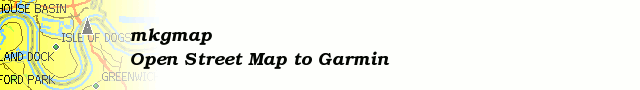# [mkgmap-dev] RFC: Consider heightmeters for routing

From Johann Gail johann.gail at gmx.de on Tue Apr 7 17:22:23 BST 2009

```> I still wonder though about the amount of cpu power needed to get that
> data - knowing that my pc calculated about 12 hours (Pentium M
> 2.26GHz, by that time 2GB RAM, harddisk with 60MB/s average write, 65
> read) on converting SRTM1" into Garmin contourlines with 10m
> seperation - for Austria only.
>
I expect it to be far less cpu intensive than calculating contour lines.
Calculating contour lines is another algorithm.

In my case I had simply to calculate the height for each node (which
would be interpolating from SRTM Raster data) and calculate then the
inclination or height difference on the street.
On long streets, which crosses several raster squares, it would be
needed to calculate the exact crossing points and calculate the height
there. As the raster resolution is afaik ~100m, this would give not too
much points. Also the temporarily generated data will be not too much,
as it adds one value to each node and each way. This will be as a rule
of thumb ~2MB per tile.

```